#Quality Digest

Featured Product
This Week in Quality Digest Live
Statistics Features
Donald J. Wheeler
Using process behavior charts in a clinical setting
Donald J. Wheeler
No one really understands kurtosis. Here’s why.
Alan Metzel
Introducing the Enhanced Perkin Tracker
Donald J. Wheeler
What you think you know may not be so
Tony Boobier
Why data leaders need to master words as well as statistics
Statistics News
New capability delivers deeper productivity insights to help manufacturers meet labor challenges
Day and a half workshop to learn, retain, and transfer GD&T knowledge across an organization
Elsmar Cove is a leading forum for quality and standards compliance
InfinityQS’ quality solutions have helped cold food and beverage manufacturers around the world optimize quality and safety
User friendly graphical user interface makes the R-based statistical engine easily accessible to anyone
Collect measurements, visual defect information, simple Go/No-Go situations from any online device
Good quality is adding an average of 11 percent to organizations’ revenue growth
Ability to subscribe with single-user minimum, floating license, and no long-term commitment
A guide for practitioners and managersBio

Statistics

## Guard Banding for Non-Capable Gages, Part 1

### Calculate the fraction of good product that will be rejected and nonconforming product that will be accepted

Published: Monday, August 23, 2021 - 12:03

All articles in this series:

IATF 16949:2016 clause 7.1.5.1.1 requires measurement systems analysis (MSA) to quantify gage and instrument variation. The deliverables of the generally accepted procedure are the repeatability or equipment variation, and the reproducibility or appraiser variation. The Automotive Industry Action Group1 adds an analytic process with which to quantify the equipment variation (repeatability) of go/no-go gages if these come in specified dimensions, or can be adjusted to selected dimensions.

The anvils of a snap gage can, for example, be set accurately to specified dimensions with Johansson gage blocks. Pin gages (also known as plug gages), on the other hand, come in small but discrete increments. If the precision to tolerance (P/T) ratio is greater than the generally accepted target, the gage cannot distinguish reliably between good and nonconforming product near the specification limits. This means nonconforming work will reach internal or external customers, while good items will be rejected, as shown in figure 1 below.

The AIAG reference (p. 182) offers the remedy of multiple measurements because the standard deviation of the average of n measurements from the same part is the gage standard deviation divided by the square root of n. The reference warns, however, “This method will, of course, be time-consuming,” but it does offer a way to keep the process running while improvements are pursued.

However, if the gage variation and process variation are known, then:
• Acceptance limits can be set to minimize the costs of the wrong decisions.
• Acceptance limits can be set to ensure that the customer receives no more than a specified fraction of nonconforming work, regardless of how poor the gage might be.

We will use the attribute gage study from the AIAG reference, which involved a non-capable process with a 0.5 process performance index, i.e., a 1.5 sigma process. The specification limits are 0.45 and 0.55, respectively, which makes the process standard deviation 0.0333. I used a gage standard deviation of 0.004 to simulate the attribute gage study, and this also results in a relatively mediocre P/T ratio (24%, and the AIAG example got 24% from the signal detection approach in its example) that will make acceptance of bad parts and rejection of good parts a problem.

Figure 1 is a MathCAD contour plot of the joint probability density function: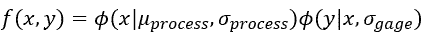where:

• ϕ is the standard normal probability density function. This need not be normal for the distribution of the part dimensions; others can be used. Measurement systems analysis generally assumes, however, that the measurements returned by the gage will follow the normal distribution. This makes sense because the range of potential measurements above and below the part’s actual dimension is a relatively narrow function of the gage’s standard deviation:
• x is the actual part dimension
• μprocess is the process mean (0.5 in the example)
• σprocess is the process standard deviation (0.0333 in the example)
• y is the measurement returned by a variables gage, or perceived by a go/no-go gage that can be set to a specified dimension or purchased in a specific dimension. This is a function of 1) the part’s actual dimension; and 2) the gage’s standard deviation σgage.
• σgage is the gage standard deviation (repeatability or equipment variation, as might be ascertained from measurement systems analysis). ϕ(y|x,σgage) is therefore the chance of getting measurement y, given that the part’s dimension is really x. The example uses 0.004.
• The specification limits are 0.45 and 0.55, respectively.

The probability that a normally distributed process will generate a part whose actual dimension or other characteristic is x is therefore, where z is the standard normal deviate:This yields four quantifiable zones of interest:
1. Good parts are accepted (correct decision). This happens when the part and the measurement are both inside the specification limits.
2. Good parts are rejected (incorrect decision). This happens when the part is in specification, but the gage returns or perceives a measurement that is not.
3. Bad parts are rejected (correct decision). The part and its measurement are both outside the specification limits.
4. Bad parts are accepted (incorrect decision). The part is out of specification, but the gage returns a measurement that is inside the limits.Figure 1: Contour plot of measurement system outcomes

The cost of the inadequate P/T ratio is therefore the fraction of the population in the top and bottom sections (good parts are rejected) times the marginal cost of the parts, plus the fraction in the left and right sections (bad parts are accepted) times the cost of external failure. If we can quantify this, we can move the acceptance limits inside the specification limits (guard band) to minimize the cost. Note also that, if we had no gage variation whatsoever, the contour plot would become a straight line for which all good parts would be accepted and all bad ones rejected.

### Example

Continue with the example shown above. Assume the marginal cost of the part (this is not in the AIAG example) is \$1, and incorporating a nonconforming one into a subcomponent at the next downstream process will incur a \$10 rework cost. The question is where to set the go/no-go gages to minimize the costs or, alternatively, where to set them to ensure that the downstream user receives no more than a certain quantity of bad items. All we need to do to answer this question is to quantify the fraction of the process output that falls into each category shown in figure 1.

1. Parts that are good and accepted

The double integral of the probability that the part is in specification, and the probability that the gage will call it in specification is shown below, where ƒ(x) is the probability density function of the process, given parameters such as the mean and process standard deviation from a normal distribution. (Remember that the measurement returned by the gage, given an actual dimension of x, will usually follow the normal distribution, and this will make our job much easier.)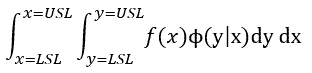Excel, and presumably other spreadsheets, have built-in functions for the cumulative normal distribution. This reduces the problem from double integration to single integration, where Φ indicates the cumulative standard normal distribution.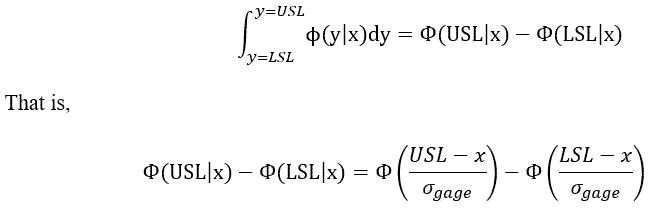Suppose, for example, that a conforming part’s measurement is 0.455; what is the chance it will be accepted?

In Excel,

=NORM.DIST(0.55,0.455,0.004,TRUE)-NORM.DIST(0.45,0.455,0.004,TRUE)

is the chance that the measurement will be less than the USL when the gage reads a part whose true dimension is 0.455 minus the chance that the measurement will be less than the LSL, which is substantial, given the gage standard deviation of 0.004. The result is 0.8944. Figure 2, as generated by StatGraphics, illustrates this, but for only one value of x. We must integrate across the entire specification limit to account for the entire range of potential part dimensions.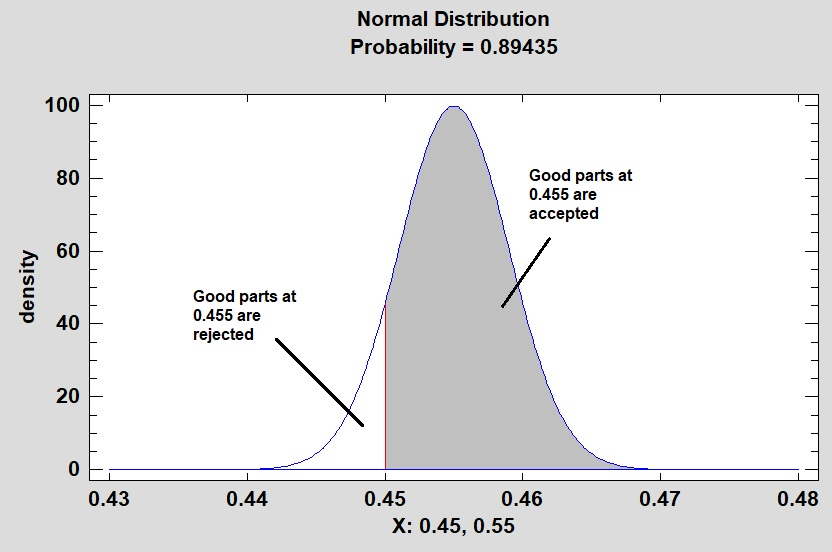Figure 2:
Probability of acceptance of a good part

The fraction of parts that are good and are accepted is therefore: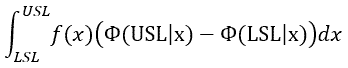2. Parts that are bad and rejected

It is extremely unlikely that parts below the LSL will be rejected by the gage at the USL, or that parts above the USL will be rejected by the gage at the LSL. To put this in perspective, the chance that a part will be rejected by the “go” (i.e., smaller) end of a plug gage because it won’t fit a hole whose diameter exceeds the upper specification limit is essentially zero. The chance that the no-go (larger) end will fit a hole smaller than the LSL, and therefore reject it for being too large, also is essentially zero. These contingencies can be disregarded. The chance that a part is bad and gets rejected is therefore: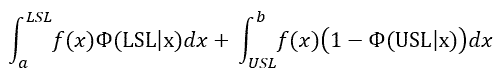The first term is the integral of the chance that the part is below the LSL times the probability it will be called bad, which is 50 percent at the LSL and increases as the part gets smaller. Integration limits a and b are far enough from the indicated specification limits to encompass the entire population of parts. That is, we would technically integrate from 0 or negative infinity to the LSL, but in this example, integration from 0.3 to 0.45 was adequate, but 0.4 to 0.45 was not. The second term is the chance of making a part larger than the USL times the chance it will be called bad, which is 50 percent at the USL and increases as the part gets larger.

3. Parts that are good and rejectedThis is the integral of the joint probability for all in-specification parts times their chances of rejection. Suppose, for example, that the part’s actual dimensions are 0.545. The chance of rejecting it is shown in figure 3, again from StatGraphics. This is for a single value of part dimension x and not for the entire range of differential values between the specification limits.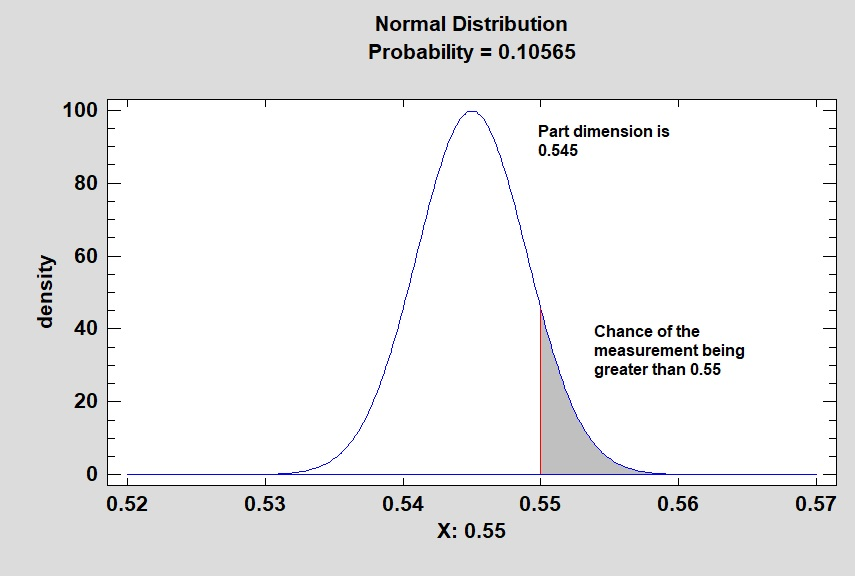Figure 3: Chance that a conforming part will be rejected

4. Parts that are bad and accepted

The chance that a part is accepted despite being out of specification is:where the first term is the integral of the chance that a part below the LSL will return a measurement greater than the LSL, and the second term is the integral of the chance that a part above the USL will return a measurement less than the USL.

In summary, where a and b are the practical counterparts of negative and positive infinity, respectively:

Equation set 1: Acceptance proportions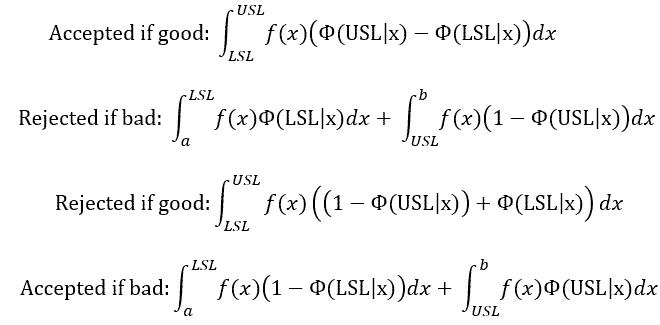Addition of these four equations leaves the following three terms that add up to the probability density function of the parts themselves, which must by necessity equal one.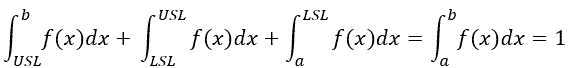This allows us to check our solutions because:
• The conforming fractions (accepted and rejected) must add to the total conforming fraction.
• The nonconforming fractions (accepted and rejected) must add to the total nonconforming fraction.
• All four fractions must add to 1.

### Example with no guard banding

Return to the original AIAG example in which the process is centered on its nominal of 0.50 but is not capable due to its 0.0333 standard deviation. The gage standard deviation is 0.004. If we accept parts whose measurements are between the specification limits of 0.45 and 0.55, how many will fall into each category? Figure 4 shows how to do this with an Excel function that is described in appendix 2.

The function Gage_Integral requires the following six arguments:
• m_process = process mean = 0.5 for this example
• s_process = process standard deviation = 0.0333
• s_gage = gage standard deviation (repeatability or equipment variation) = 0.004
• a = lower limit of integration = 0.3, 0.4 did not account for all the nonconforming parts below the LSL
• b = upper limit of integration = 0.7, 0.6 did not account for all the nonconforming work above the USL
• accept = acceptance limit, which is the specification limit without guard banding
• left = TRUE means to assess the probability of acceptance to the left of the acceptance limit, and FALSE means to assess the probability of acceptance to the right of the acceptance limit. This relates to the gage and not to the process.

The next question is how to put this into practice because Excel does not contain a built-in integration function. My experience is that Romberg integration2 is the most computationally effective and accurate method (see appendix 2 for the details), and can be deployed as an Excel function via Visual Basic for Applications (VBA). This will provide readers with an extremely powerful tool with which they can either 1) set acceptance limits to minimize cost; or 2) assure a customer that their sampling procedure will deliver no more than a specified nonconformance level. Appendix 1 provides more detail about Romberg integration, and appendix 2 contains the Visual Basic for Applications function Gage_Integral for Excel.

Figure 4 shows the outcome of this exercise:Figure 4: Example with no guard banding

The total good and bad portions were calculated by using Excel’s normal distribution function for the specification limits, while using the given process mean and standard deviation. The Accepted if Good and Rejected if Good fractions must add up to the total good portion, and Accepted if Bad and Rejected if Bad must add up to the total bad portion. In addition, all four categories must add to 1 to account for 100 percent of the population. Here is how each category is calculated.

1. Accepted if good = the integral from the LSL to the process mean using the fraction to the right of LAL (lower acceptance limit) = LSL plus the integral from the mean to the USL using the fraction to the left of the upper acceptance limit (UAL) = USL.
2. Rejected if good = the integral from the LSL to the USL counting the fraction to the left of LAL = LSL (i.e., the gage thinks the measurement is below the LAL) plus the same integral counting the fraction to the right of UAL = USL, i.e., the gage thinks the measurement is above the UAL.
3. Accepted if bad = the integral from a = 0.3 to the LSL and counting the fraction to the right of LAL = LSL, i.e., the gage thinks the parts are larger than the LAL, plus the integral from USL to b = 0.7 and counting the fraction to the left of UAL = USL, i.e., the gage thinks the parts are smaller than the UAL.
4. Rejected if bad = the integral from a to the LSL and counting the fraction to the left of the LAL, i.e., the gage says they are too small, plus the integral from the USL to b and counting the fraction to the right of the UAL, i.e., the gage says they are too large.

How were the integration limits of a = 0.3 and 0.7 determined for assessing the nonconforming fractions? I first tried 0.4 and 0.6, respectively, but the sum of the fractions for nonconforming parts (accepted and rejected) did not add up to the total nonconforming fraction. This means some of the area for the double integration was omitted. Using 0.3 and 0.7, however, worked. This is why it is important to compare the totals. As a further check on the VBA function, I did the same calculations in MathCAD, which does have a built-in integration function, and got the same results.

Part one of this two-part series has shown that if we are given 1) the probability distribution of the process, which need not be normal; and 2) the variation of the gage, we can calculate the fraction of good product that will be rejected and nonconforming product that will be accepted. Part two will show how to optimize acceptance limits to either minimize the total cost of wrong decisions, or assure the customer that the process will deliver no more than a specified fraction of nonconforming work. This is known as guard banding.

References
1. Automotive Industry Action Group. Measurement Systems Analysis, 4th Edition, Section C: Attribute Measurement Systems Study, 2010.
2. Hornbeck, Robert W. Numerical Methods. Quantum Publishers, New York, 1975. pp. 150–154.

### Appendix 1: Romberg integration

Consider the integral of any function from a to b.Romberg integration then defines for the mth iteration, and T refers to a trapezoidal rule estimate of the integral:For the first iteration, noting that the summation is inoperative (we cannot sum from j = 1 to 0), this is simply the area of the trapezoid with base (b-a), i.e., the distance between the limits of integration, and sides with heights ƒ(a) and ƒ(b).Subsequent calculations can be done recursively where: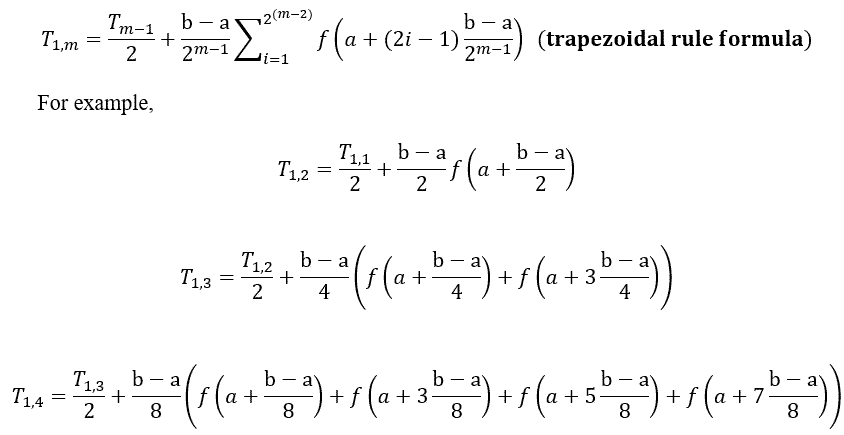The trapezoidal rule estimates become more accurate with more iterations, but the reference also cites an error term that must be eliminated. An extrapolation procedure is used for this purpose, and it converges to the correct answer more quickly than the trapezoidal rule values would alone. Note that n is 2 or more because the values for n = 1 come from the trapezoidal rule.As an example, noting that the two terms in the parenthesis are available from the trapezoidal rule,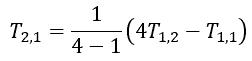Then the objective is to proceed as shown in figure 5 to get the value in the lower right-hand corner. Of course, the table may have a lot more rows and columns than illustrated here, e.g., there may be 32 rows and the final result T32,1 although a simple example given by Hornbeck arrived at the correct answer at T3,1. Extrapolation is carried out at each stage for n = 1 to m and k = 1 to m–1; as shown below, this is for n = 1 to 4

 T1,1 T1,2 T2,1 T1,3 T2,2 T3,1 T1,4 T2,3 T3,2 T4,1

Figure 5: Romberg integration

The stopping rule is, then, for a selected absolute or relative error ϵ:Example

The integral of x2 from 0 to 3 is x3/3 = 9. If we use Romberg integration:This simple example required only two iterations to reach the correct answer. The gage integration function seems to require on the order of 20 to 24 when using the normal probability density function for the part characteristic and cumulative normal distribution for the measurement.

### Appendix 2: Gage_Integral Function for Excel

Public equation As String
' Make the function double precision
Public Function Gage_Integral(m_process As Single, s_process As Single, s_gage As Single, a As Single, b As Single, accept As Single, left As Boolean) As Double
' where the arguments are in the following order
' m_process = process mean
' s_process = process standard deviation
' s_gage = gage standard deviation
' a = lower limit of integration
' b = upper limit of integration
' the distance between a and the LSL, and the USL to b, must be great enough
' to account for the entire probability distribution of the parts and their possible measurements
' accept = lower or upper
' left = true means to calculate the cumulative distribution to the left of the stated acceptance limit. False invokes the right tail.
' The function is then uses as follows.
' =Gage_Integration(m_process,s_process,s_gage,a,b,accept)
' recall that the specification limits are taken care of by the limits of integration
'Dimension T as double precision as well
Dim Sum As Double, T(1000, 1000) As Double
m = 1
' First trapezoidal rule value
T(1, 1) = ((b - a) / 2) * (g(a, m_process, s_process, s_gage, accept, left) + g(b, m_process, s_process, s_gage, accept, left))
' where g is the joint probability that the part is a given measurement times the chance it will be accepted or rejected
Iterate1:
m = m + 1
Sum = 0
Delta = (b - a) / (2 ^ (m - 1))
For i = 1 To 2 ^ (m - 2)
Sum = Sum + g(a + (2 * i - 1) * Delta, m_process, s_process, s_gage, accept, left)
Next i
Sum = Sum * (b - a) / (2 ^ (m - 1))
T(1, m) = T(1, m - 1) / 2 + Sum
' Romberg extrapolation
For l = 2 To m
For k = 1 To m - 1
T(l, k) = ((4 ^ (l - 1)) * T(l - 1, k + 1) - T(l - 1, k)) / (4 ^ (l - 1) - 1)
Next k
Next l
' Compare T(L,1) to T(L-1,1)
If m < 4 Then GoTo Iterate1 'Don't test for convergence before 4 iterations
If T(m, 1) < 0.00000001 Then GoTo SkipTest 'The integral is really zero
Test = Abs((T(m, 1) - T(m - 1, 1)) / T(m, 1))
If Test > 0.00000001 Then GoTo Iterate1 'Smaller values result in higher precision but more computation time
SkipTest:
Gage_Integral = T(m, 1)
End Function

Function g(x, m_process, s_process, s_gage, accept, left) As Double
With Application.WorksheetFunction
If left = True Then
' Find the cumulative probability density to the left of the acceptance limit
' We can use distributions other than the normal for the first factor, if the process
' does not conform to a bell curve.
g = .NormDist(x, m_process, s_process, False) * .NormSDist((accept - x) / s_gage)
' Otherwise find the cumulative probability to the right of the acceptance limit
Else: g = .NormDist(x, m_process, s_process, False) * .NormSDist((x - accept) / s_gage)
End If
End With
End Function

This can be added to an Excel spreadsheet with the Visual Basic for Applications option under the Developer menu. (The latter must be added to the ribbon using the options.) The spreadsheet must be saved as a macro-enabled spreadsheet (.xlsm).

### Appendix 3. Non-normal distributions

Figure 6 from MathCAD shows a process whose critical-to-quality characteristic, such as a trace contaminant, pollutant, or impurity, follows a gamma distribution whose shape parameter is 2 and whose scale parameter is 1. The upper specification limit is 6 ppm, and there is no LSL because we do not care how little of the undesirable characteristic we get. The instrument has a standard deviation of 0.1 ppm.Figure 6: Joint probability density function, non-normal process and normal gage

This situation is actually simpler than the one with the normally distributed characteristic and its bilateral specification limits, as we need worry only about the USL. Excel does have a built-in function, GAMMA.DIST, that will return the probability density function for the gamma distribution, which offers the ability to calculate similarly a tightened acceptance limit.

Part two of this series will show how to optimize the acceptance limits to either minimize the cost of wrong decisions, or assure the customer that it will receive no more than a specified fraction of nonconforming work.

### Discuss